# AI: Using the Compact Genetic Algorithm to Compute Square Roots in C#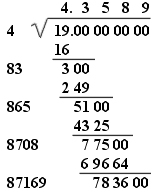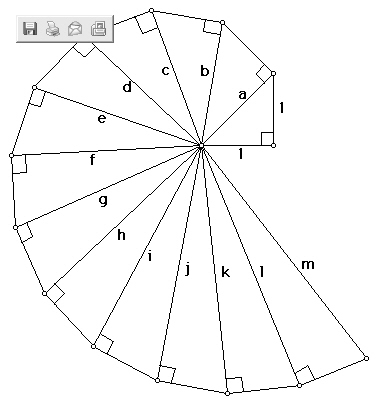Introduction

There are many ways to compute square roots.  Some of these include using approximation, using recursive reciprocals, or just using simple geometry.  In our example we will show you how to compute a square root using a genetic algorithm and at the same time introduce a fast and compact GA called the Compact Genetic Algorithm.

The Compact Genetic Algorithm (cGA) is similar to the PBIL (Population Based Incremental learning) but requires fewer steps, fewer parameters and less of a gene sample.  The following steps describes the Compact Genetic Algorithm.

1. Initialize a probability vector  p[n]  to a probability of 1/2 for all  vector positions
(for i=0 to length) p[i] = 0.5

2. Generate two individual genomes (x and y) from the probability vector with each genome containing a string of 1's and 0's of the same length of the probability vector.  The probability that the position of the genome contains a one is p[position] and the probability that it contains a zero is  (1 - p[position]).
x = GenerateGenome (p);   y = GenerateGenome (p)

3. let the two genes (x and y )  compete:
winner, loser =  EvaluateFitness (x, y)

4. update the probability vector p[n]  using the winner of x and y.  This will cause the probability vector to move toward the winning gene.
if (winner[i] != loser[i]) then
if winner[i] == 1 then p[i] = p[i] + 1/population_size
if winner[i] = 0 then p[i] = p[i] - 1/population_size

5. Check to see if the probability vector has converged, if not go back to step 2
for i = 1 to length;    if p[i] > 0 && p[i] < 1 then goto step_2;

6. p represents the final converged solution

Note that their are only two variables in the compact genetic algorithm:  the length of the genome and the population size.  Unlike the Simple Genetic Algoirthm,  no actual population is required, just the two genomes x and y.   The population_size variable simulates the actual population size by increasing or decreasing the learning rate (similar to PBIL).  Admittedly, you seem to lose some of the magic of regular genetic algorithms, when you don't have a population size, but the compact genetic algorithm seems to have some performance significance so we will demonstrate it here.

Design

The Design for the Compact Algorithm consists of mainly two classes:  a Population class that implements the algorithm, and a BinaryChromosome class that generates the random genomes from the probability vector and calculates the fitness function.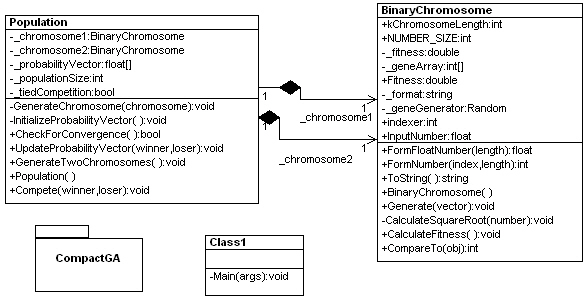Figure 2 - UML Design of Compact Genetic Algorithm reverse engineered using WithClass

Code

The code simply goes step by step through the algorithm and repeats the algorithm until convergence is reached.  The code takes advantage of object-oriented design in figure 2 to place functionality relating to the genome in the BinaryChromosome class and functionality relating to the competition between genomes in the population in the Population class.   The code implementing the compact genetic algorithm is shown in Listing 1 below.

Listing 1 - Implementing the cGA Algorithm in C#

// Step 1: Initialization of the probability vector happens here
Population population = new Population();
BinaryChromosome winner =
new BinaryChromosome();
BinaryChromosome loser =
new BinaryChromosome();
int generation = 0;
// step 5: checks for convergence to the probability vector
// each time through the loop
while (population.CheckForConvergence() == false)
{
if (generation == 20000)
{
population.UpdateProbabilityVector(winner, loser);
}
// step 2: Generate two genomes from the current probability vector
population.GenerateTwoChromosomes();
// step 3: Allow the two chromosomes to compete and
// determine a winner and a loser from the fitness
population.Compete(out winner, out loser);
// step 4: Update the probability vector based upon
// the winning genome
population.UpdateProbabilityVector(winner, loser);
if (generation > 1000000)
break;
generation++;
}
// step 6:
// write out the final genome with the highest fitness after
// convergence or the generations go past 1,000,000
population.UpdateProbabilityVector(winner, loser);
Console.WriteLine("The Square Root of {0} Is {1} at generation #{2}",
BinaryChromosome.InputNumber, winner, generation);
Console.WriteLine();
}

As with all Genetic Algorithms, the success of the algorithm depends strictly on the fitness function you provide to determine the fitness of the chromosomes for each generation.  In cGA,  all our genomes are represented as 1's and 0's.   In order to compute a genome containing a representation of the best square root,  we need to translate the 1's and 0's binary representation into a floating point number.  In this example we used a 30 bit number.  The high order 15 bits represents the integer part of the floating point number, and the low order 15 bits represents the decimal portion of the number.  Therefore, the best number we can represent is a 215  integer (32768)  with a decimal precision of  215.  So how do we represent a decimal number in binary?  In base 10 each digit is represented in powers of 10.   The number 3.5 would be represented as

3 x 100  + 5 x 10-1

In binary, we represent the number 3.5 as a sum of powers of 2:

1 x 21 + 1 x 20 + 1 x 2-1

The two halves of the binary number are shown below:

3                      1/2

000000000000011 100000000000000

If we wanted to represent 3.75,   we would tack on an extra 1/4,  adding an additional  1 x 2-2

3                      3/4

000000000000011 110000000000000

Listing 2 shows how we can convert our binary genome string into a floating point value.  The method loops through each character in the binary string (either a 1 or 0),  shifts the number 1 to the left and adds it to the existing number.  This shifting and adding will produce the integer equivalent of a 30 bit binary number scaled by 215.  To make it floating point, we just need to divide the final integer by 215  or 32768.

Listing 2 - Converting a binary string to a floating point value

public float FormFloatNumber(int length)
{
float result = 0;
int num = 0;
// form the integer number from the binary string
for (int i = 0; i < length; i++)
{
num = num << 1;
num = num + _geneArray[(length - i) - 1];
}
// scale to the floating point value
result = (float)num;
result = result / (1 << kChromosomeLength/2);
return result;
}

Fitness Function

The fitness function takes the genome in its binary string form and converts it to a floating point value by calling FormFloatNumber.  It then squares the number and compares it to the value from which we are trying to obtain the square root.  Listing 3 shows the fitness function for our binary genome:

Listing 3 - Fitness function to check the Square Root

void CalculateSquareRootFitness(float number)
{
// first get the floating point representation of the binary genome
// approximating the square root
float root = FormFloatNumber(kChromosomeLength);
// calculate the fitness by squaring the genome value and subtracting the desired value
_fitness = 1.0f/Math.Abs((root * root) - number);
}

When we run the Compact Genetic Algorithm on a number for which we desire the square root, we find that although it generally takes many generations to come up with the solution, we usually get a fairly accurate result.   Figure 3 shows the result of running the Compact Genetic Algorithm on several different numbers to attain a square root.  The output shows the inputted number,  its square root,  the fitness generated(directly after the arrow) and the number of generations it took to converge.  Note that some generations went all the way to 1,000,000.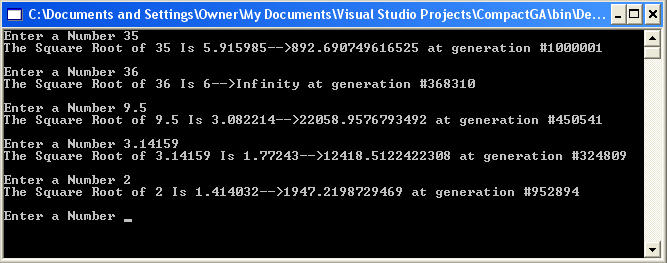Figure 3 - Output of our C# Compact Genetic Algorithm for computing Square Roots

Getting the Nth Root

We can actually take this functionality a step further.  By slightly altering the fitness function, we can compute solutions for any root.  We simply multiply the genome floating point representation by the number of times in the exponent and compare to the inputted number.  For example if we want the 4th root of 5,  we  can test the genome value as a possible solution, by multiplying it four times and comparing the final value to 5.  Listing 4 shows the fitness function for the nth root test.

void CalculateNthRootFitness(float number, int exponent)
{
// form the floating point value from the binary string of the genome
float root = FormFloatNumber(kChromosomeLength);
float product = 1.0f;
// calculate the product by raising the root to the nth power
// (Essentially by multiplying it n times)
for (int i = 0; i < exponent; i++)
{
product = product * root;
}
// compare the value of the product to the actual input number
// and compute a fitness
_fitness = 1.0f/Math.Abs(product - number);
}

Figure 4 shows the results of inputting different numbers and looking for the nth root.  Note that some final genome values have lower fitnesses than others.  The cubed root of 40 comes up with a solution of 3.419922 with a fitness of only 949,   where as the 5th root of 32 produces a solution of 2 with a fitness of Infinity.  A fitness of Infinity tells us that our genome's root converged  right on the nose.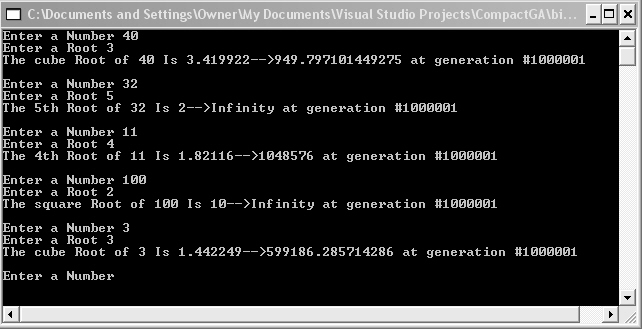Figure 4 - Computing the nth root using the Compact Genetic Algorithm

Conclusion

Like PBIL,  the Compact Genetic Algorithm uses probability vectors to come up with genomes and converge upon the best solution to a particular fitness function.  One thing I like about the Compact Genetic Algorithm is that it is fast, and only requires two genomes at any one time.  Unfortunately, cGA seems to lose diversity by not maintaining a population of several genomes, and perhaps narrows in on only one solution.  This makes the algorithm very susceptible to local minimum solutions.  However, it does seem to work fairly well for our example, so it is worth exploring.  Enjoy tinkering with the C#ompact Genetic Algorithm!

References

The Compact Genetic Algorithm ,  George R. Harik, Fernando G. Lobo, and David E. Goldberg.

AI: Population Based Incremental Learning in C# and .NET,  Michael Gold

Recommended Ebook